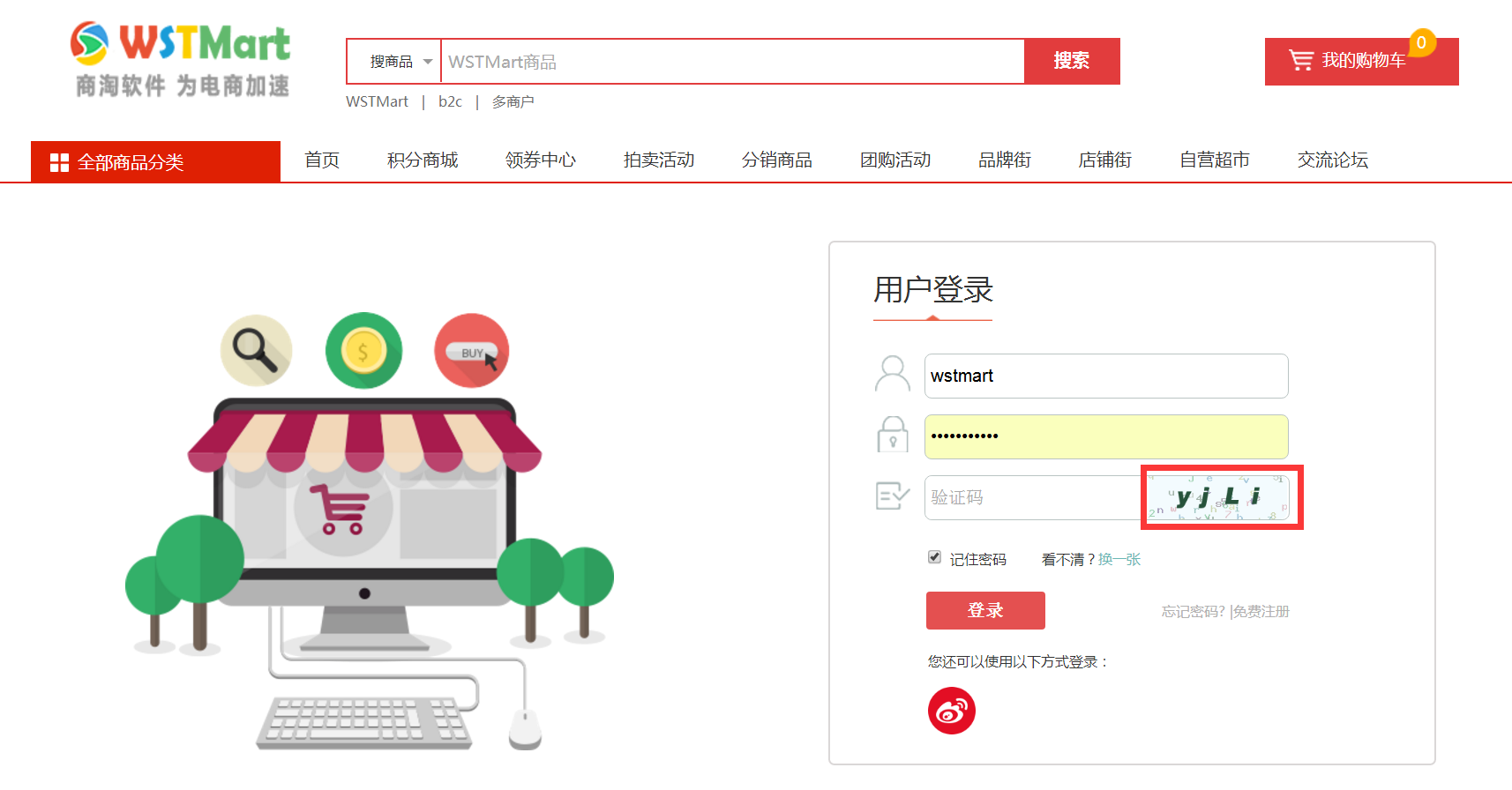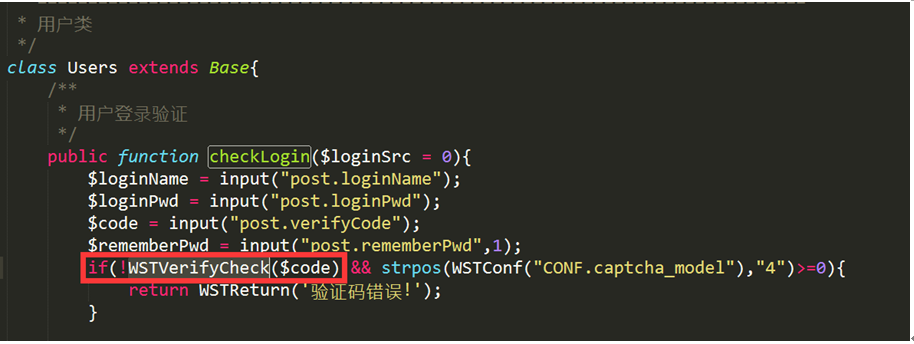現在不管使用什麼系統，都需要進行各式各樣的身份驗證，在各種專案中驗證碼是必須會用到的，驗證碼從誕生開始就是為了區別出到底是人類操作，還是機操作。它在一定程度上可以提高網站的安全性，可以防止惡意註冊及惡意破解密碼。/**

* 獲取驗證碼

*/

public function getVerify(){

WSTVerify();

}

/**

* 生成驗證碼[在thinkphp5中可以靈活配置引數生成驗證碼]

*/

function WSTVerify(){

\$Verify = new \verify\Verify();

\$Verify->length  = 4; //驗證碼位數

//\$Verify->expire =1800;

//\$Verify->useZh= false;//中文驗證碼字串

//\$Verify->fontSize= 15;  //驗證碼字型大小(px)

//\$Verify->useCurve= true;  //是否畫混淆曲線

//\$Verify->useNoise= true;  //是否新增雜點

//\$Verify->imageH= true;  //是否新增雜點

//\$Verify->imageW= true;  //是否新增雜點

//\$Verify->reset= true;  //驗證成功後是否重置

\$Verify->entry();

}

/**

* 核對驗證碼

*/

functionWSTVerifyCheck(\$code){

\$verify = new \verify\Verify();//建立驗證器

return \$verify->check(\$code);//呼叫check方法進行驗證

}